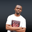Related Tags

d programing
arrays
communitycreator

# How to slice an array in DNDUKWE CHIDERA K.

### What is slicing?

A slice operation involves taking out some part of an array and returning the other part.

### Slicing a D array

Slicing an array in D implies specifying a subarray of an array. When an array is sliced, it does not mean that data was copied to another variable. It only creates another reference to such an array.

#### How to do a slice

• First, we create the array that we want to slice.
int [] let_slice = [ 5,6,7,8,9];

• We indicate the indexes from which to start our slice and at which to end it. We separate these indexes by triple dots. We slice array let_slice from the first to the fourth index position.
let_slice[1...4]


If we output this, we’ll see what it contains.

### Example

In the code below, we’ll return the slice value of the array let_slice.

import std.stdio;

void main() {

//an array of int type ;
int[] let_slice = [ 1,3,4,5,6,7];
write("These are the values between index 1 and 4: ");
//slicing the array
writeln(let_slice[1..4]);
}
Array slicing

### Explanation

• Line 1: We import the stdio package.

• Line 3: We start the main function.

• Line 6: We declare an array of int data type.

• Line 7: We write a note to the output.

• Line 9: We print the outcome of slicing the array let_slice.

RELATED TAGS

d programing
arrays
communitycreator

CONTRIBUTORNDUKWE CHIDERA K.
RELATED COURSES

View all Courses

Keep Exploring

Learn in-demand tech skills in half the time